Next: The Common Collector Amplifier Up: The Common Emitter Amplifier Previous: The Basic CE Amplifier

## CE Amplifier with Emitter Resistor

The CE amplifier is often constructed with an emitter resistor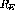as shown in figure 5.9. This resistor provides a form of negative feedback that can be used to stabilize both the DC operating point and the AC gain. It can be shown that the voltage transfer function across the transistor is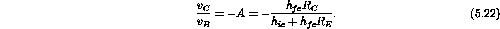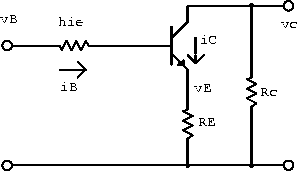Figure 5.9:  CE amplifier with emitter resistor.

If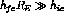, the gain becomes independent of the hybrid parameters:Because it is unaffected by variations in the hybrid parameters, this result is valid even for a large-amplitude signal.

The input resistance can be shown to be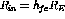. Since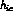is small, it can usually be neglected whenever the emitter impedance is more than a few hundred ohms.

For small input signals it is often desirable to retain the large voltage gain of the basic CE amplifier even though an emitter resistor is used for DC stability. This can be done if a large capacitoris used to bypass the AC signal around the emitter resistor.shorts out the emitter bias resistorfor AC signals. The magnitude of the resulting transfer function is similar to a high-pass filter, withsetting the gain (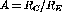) at low frequencies and the capacitor maximizing the gain (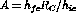) at high frequencies.

Example: Determine values for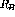and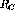that will yield the operating point shown in the circuit in figure 5.10 (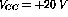) under the assumption that:

1.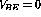.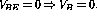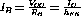.

Therefore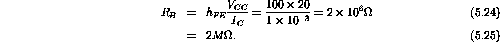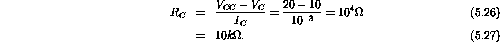2.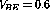V.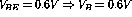.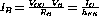.

Therefore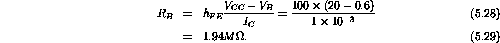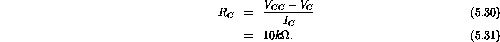3. Repeat both calculations for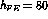.

For,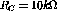remains unchanged.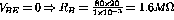.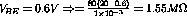.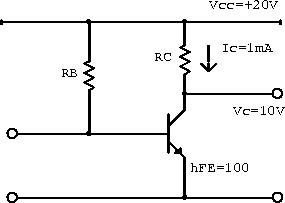Figure 5.10:  Example transistor circuit with a single bias transistor.

Example:
1. If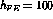, determine an expression for the base-biasing resistorthat will result in a DC operating point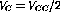for the circuit in figure 5.11.

Now. The voltage drop across the bias resistor is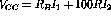. Charge conservation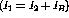gives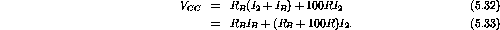The voltage at the base is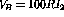. Neglecting the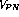voltage drop gives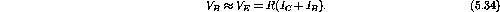EliminOAating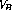gives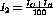. Using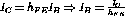gives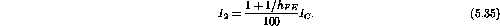Substituting equation 5.35 into equation 5.33 gives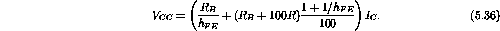The voltage drop across the collector resistor is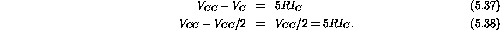Therefore2. If the circuit is built with thejust found, what will be the operating point if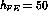?

If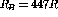and,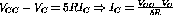.

Substitution into equation 5.36 gives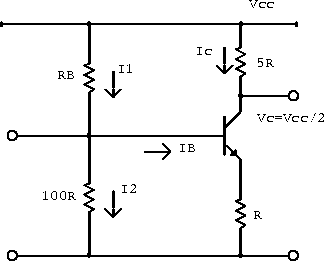Figure 5.11:  Example transistor circuit with two bias resistors.Next: The Common Collector Amplifier Up: The Common Emitter Amplifier Previous: The Basic CE Amplifier

Doug Gingrich
Tue Jul 13 16:55:15 EDT 1999